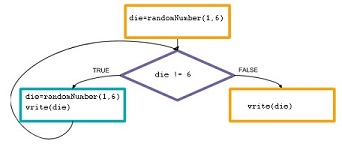# While loop

Category:Control

## While block

Category: Control

Executes a block of statements while the specified condition is true.

Apps sometimes need to repeatedly execute some code while something is true. The while loop uses a boolean condition to repeatedly run a block of code. It will check the expression, and if it is true it runs the block of code contained within the curly braces. This process of checking the condition and running the block of code is repeated as long as the boolean condition remains true. Once the boolean expression becomes false it will stop.

A while block requires you to define an expression that evaluates to true or false. Just as in arithmetic there are some operators you can use to write expressions that evaluate to a number, programming languages also have a comparison operators (< <= == > >= !=) and boolean operators (&& || !) that let you write expressions that evaluate to true or false.

### Examples

 ```// Keep rolling a die while you do not roll a 6. (event-controlled loop) var die=randomNumber(1,6); while (die !=6) { write(die); die=randomNumber(1,6); } write(die); ``````// Roll a die 5 times. (counter-controlled loop)
var count=1;
while (count<=5) {
write(randomNumber(1,6));
count=count+1;
}
```

Example: Prompt and Loop Prompt the user for exam scores and find the average.

```// Prompt the user for exam scores and find the average.
var examGrade = promptNum("Enter an exam score from 0 to 100:");
var sum=0;
var count=0;
count=count+1;
examGrade = promptNum("Enter an exam score from 0 to 100:");
}
if (count>0) {
write("Average="+(sum/count));
}
else {
write("No valid exam scores entered");
}
```

### Syntax

```while (condition) {
statement
}
```

### Parameters

Name Type Required? Description
condition boolean expression Yes An expression that evaluates to true or false. Comparison operators include < <= == > >= !=. Boolean operators include &&
statement App Lab statement(s) Yes Any valid App Lab statements.

No return value.

### Tips

• Unlike an event handler, an while statement does not constantly monitor your program checking the condition to see if it's true or false. A while statement is an instruction just like any other that gets executed line by line in order from top to bottom.
• Ensure the while loop condition eventually evaluates to false to prevent an infinite loop.
• If the condition in the while loop is false initially, the loop is never entered and execution continues with the statement following the loop.
• If the problem phrases the iteration as an until (roll a die until you roll a 6), the while condition usually uses a negation, !(die==6).
• It is sometimes helpful to use the debugging tools (setting breakpoints, inspecting variable values, and stepping) to help correct a loop error.

Found a bug in the documentation? Let us know at documentation@code.org

## Syntax

```while(  ){ // code }
```

Found a bug in the documentation? Let us know at documentation@code.org